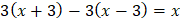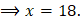# A man was asked to state his age in years. His reply was, “Take my age 3 years hence, multiply it by 3 and then subtract 3 times my age 3 years ago and you will know how old I am.” What is the age of the man? (a) 18 yrs. (b) 20 yrs. (c) 24 yrs. (d) 32 yrs.

## Question ID - 54419 :- A man was asked to state his age in years. His reply was, “Take my age 3 years hence, multiply it by 3 and then subtract 3 times my age 3 years ago and you will know how old I am.” What is the age of the man? (a) 18 yrs. (b) 20 yrs. (c) 24 yrs. (d) 32 yrs.

3537

Let the present age of the man beyrs. Then,The present age of man is 18 yrs.

Next Question :
 The sum of the ages of Janyat, Prem and Paras is 93 years. Ten years ago, the ratio of their ages was 2:3:4. What is the present age of Paras? (a) 24 yrs. (b) 28 yrs. (c) 32 yrs. (d) 38 yrs.